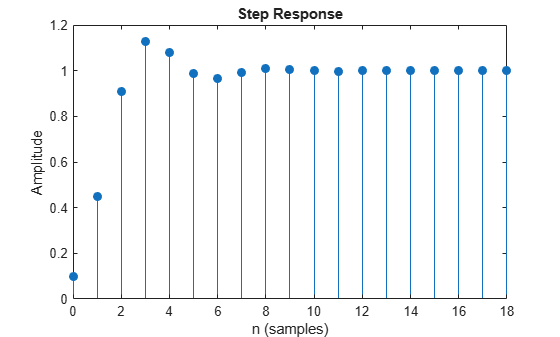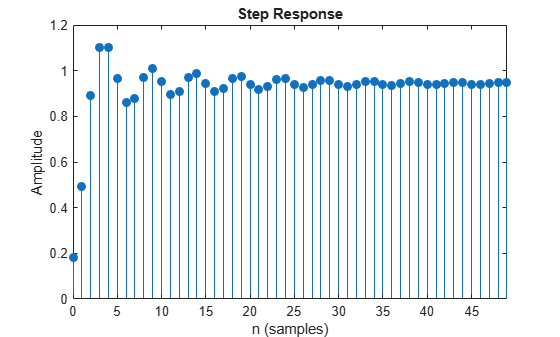Documentation

# stepz

Step response of digital filter

## Syntax

```[h,t] = stepz(b,a) [h,t] = stepz(sos) [h,t] = stepz(d) [h,t] = stepz(...,n) [h,t] = stepz(...,n,fs) stepz(...) ```

## Description

`[h,t] = stepz(b,a)` returns the step response of the filter with numerator coefficients, `b`, and denominator coefficients, `a`. `stepz` chooses the number of samples and returns the response in the column vector `h` and sample times in the column vector `t` (where `t = [0:n-1]'`, and `n` = `length(t)` is computed automatically).

`[h,t] = stepz(sos)` returns the step response of the second order sections matrix, `sos`. `sos` is a K-by-6 matrix, where the number of sections, K, must be greater than or equal to 2. If the number of sections is less than 2, `stepz` considers the input to be the numerator vector, `b`. Each row of `sos` corresponds to the coefficients of a second-order (biquad) filter. The ith row of the `sos` matrix corresponds to ```[bi(1) bi(2) bi(3) ai(1) ai(2) ai(3)]```.

`[h,t] = stepz(d)` returns the step response of the digital filter, `d`. Use `designfilt` to generate `d` based on frequency-response specifications.

`[h,t] = stepz(...,n)` computes the first n samples of the step response when n is an integer (`t` = `[0:n-1]'`). If `n` is a vector of integers, the step response is computed only at those integer values with 0 denoting the time origin.

`[h,t] = stepz(...,n,fs)` computes n samples and produces a vector `t` of length `n` so that the samples are spaced `1/fs` units apart. `fs` is assumed to be in Hz.

`stepz(...)` with no output arguments plots the step response of the filter. If you input the filter coefficients or second order sections matrix, the current figure window is used. If you input a `digitalFilter`, the step response is displayed in FVTool.

### Note

If the input to `stepz` is single precision, the step response is calculated using single-precision arithmetic. The output, `h`, is single precision.

## Examples

collapse all

Create a third-order Butterworth filter with normalized half-power frequency $0.4\pi$ rad/sample. Display its step response.

```[b,a] = butter(3,0.4); stepz(b,a) grid```Create an identical filter using `designfilt` and display its step response using `fvtool`.

```d = designfilt('lowpassiir','FilterOrder',3,'HalfPowerFrequency',0.4); stepz(d)```Design a fourth-order lowpass elliptic filter with normalized passband frequency $0.4\pi$ rad/sample. Specify a passband ripple of 0.5 dB and a stopband attenuation of 20 dB. Plot the first 50 samples of the filter's step response.

```[b,a] = ellip(4,0.5,20,0.4); stepz(b,a,50) grid```Create the same filter using `designfilt` and display its step response using `fvtool`.

```d = designfilt('lowpassiir','FilterOrder',4,'PassbandFrequency',0.4, ... 'PassbandRipple',0.5,'StopbandAttenuation',20, ... 'DesignMethod','ellip'); stepz(d,50)```## Algorithms

`stepz` filters a length `n` step sequence using

```filter(b,a,ones(1,n)) ```

and plots the results using `stem`.

To compute `n` in the auto-length case, `stepz` either uses `n = length(b)` for the FIR case or first finds the poles using `p = roots(a)`, if `length(a)` is greater than 1`.`

If the filter is unstable, `n` is chosen to be the point at which the term from the largest pole reaches 106 times its original value.

If the filter is stable, `n` is chosen to be the point at which the term due to the largest amplitude pole is 5 × 10–5 of its original amplitude.

If the filter is oscillatory (poles on the unit circle only), `stepz` computes five periods of the slowest oscillation.

If the filter has both oscillatory and damped terms, `n` is chosen to equal five periods of the slowest oscillation or the point at which the term due to the pole of largest nonunit amplitude is 5 × 10–5 times its original amplitude, whichever is greater.

`stepz` also allows for delays in the numerator polynomial. The number of delays is incorporated into the computation for the number of samples.